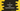# Insertion sort implementation in C : Sorting an integer array### Sorting an integer array using insertion sort using C programming language :

Insertion sort is one of the simplest sorting algorithm. It iterates through the items one at a time and keeps building the final sorted array. For example, for an array of 5 elements, it first sorts the first two elements , then first three elements , next first four elements and so on.

### Time complexity of insertion sort :

Best case : Best case if the array is already sorted. In this case, the insertion sort has O(n) time complexity. Worst case : Worst case if the array is sorted in reverse order. In this case the time complexity is O(n2).

For smaller sized array, insertion sort is one of the fastest algorithm. It is even faster than Quicksort.

### Explanation :

1. Our input array is 3,7,5,6,9
2. Start from the second element and compare it with all left elements from it. First, we compared 7 with 3, since 3 < 7 move to next step.
3. Now for third element 5, compare it with all left elements. Since, 7>5, swap it. Again 5>3 so keep it same.
4. For the fourth element 6, do the same thing and place it between 5 and 7.
5. For the last element 9, since it is greater than its left element, keep the array same.

### C program of insertion sort :

``````#include

//8
void insertionSort(int *array,int length){
//9
int i,j,temp;

//10
for(i=1;i<length;i++){ //11 j = i; //12 while(j>0)
{
//13
if(array[j-1] > array[j])
{
int temp = array[j-1];
array[j-1] = array[j];
array[j] = temp;
}else{
break;
}

//14
j--;
}
}
}

int main(){
//1
int total;
int i;

//2
printf("Enter total elements of the array : ");
scanf("%d", &total);

//3
int array[total];

//4
for(i=0;i<total;i++){
printf("Enter element %d : ",(i+1));
scanf("%d",&array[i]);
}

//5
printf("Array before sorting : \n");
for(i=0;i<total;i++){
printf("%d ",array[i]);
}

//6
insertionSort(array,total);

//7
printf("\nArray after sorting : \n");
for(i=0;i<total;i++){
printf("%d ",array[i]);
}
}``````

### Explanation :

The commented numbers in the above program denote the step number below :

1. Create one integer total to store the total elements and one integer i to use in the for loop.
2. Ask the user to total number of elements and store it in total variable.
3. Create one integer array of size total.
4. Run one for loop to read all elements and store it in the array.
5. Print all the elements of the array.
6. Call the method insertionSort() to sort the array.
7. After the sort is completed, print out the array elements again.
8. insertionSort takes the reference of the array and size of the array as input. It sorts the elements of the given array using insertion sort.
9. Integer i and j to use in the loop. Variable temp to store one element temporarily while swapping.
10. Run one for loop to scan from the first element to the last element of the array.
11. Set the current value of i to j.
12. Now run one while loop to scan all the left elements of the current element.
13. If the current element is less than the left element, swap both. Then again compare it with its other left elements. If the left element of the current element is smaller, break from the while loop.
14. j is decrementing by 1 each time to check for other left elements.

### Sample Output :

``````Enter total elements of the array : 5
Enter element 1 : 5
Enter element 2 : 4
Enter element 3 : 3
Enter element 4 : 2
Enter element 5 : 1
Array before sorting :
5 4 3 2 1
Array after sorting :
1 2 3 4 5

Enter total elements of the array : 5
Enter element 1 : 100
Enter element 2 : 84
Enter element 3 : 13
Enter element 4 : 90
Enter element 5 : 1
Array before sorting :
100 84 13 90 1
Array after sorting :
1 13 84 90 100``````